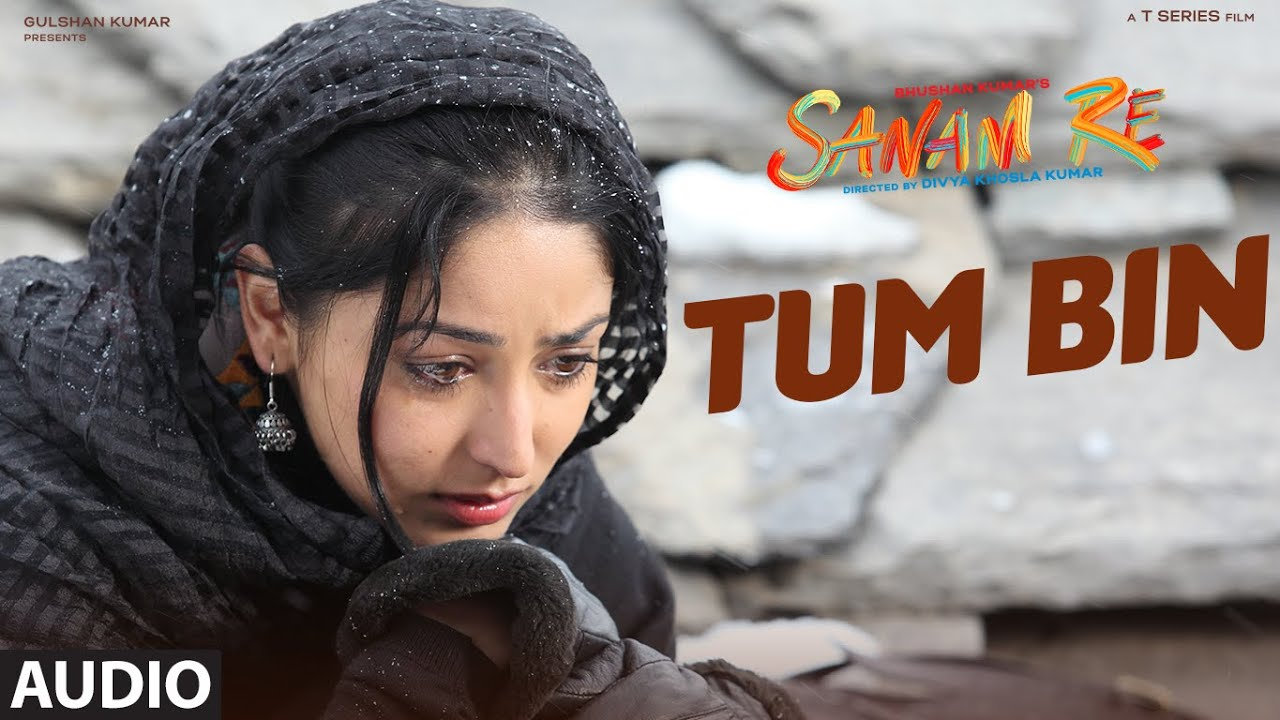# Tum Bin Sanam Re Piano Notes – Sanam Re

## Tum Bin Sanam Re Piano Notes , Keyboard Notations , Casio NotationsSong : Tum Bin
Singer : Shreya Ghoshal
Lyric : Rashmi Virag
Casting : Pulkit Samrat, Yami Gautam, Divya Khosla Kumar
Music on : T-Series
Download : Tum Bin – Sanam Re

For Buying Full Notes Send Email – [email protected]

Kuch pal to thehar jaao na
G+G+A+D+D+E+D+C+C+E+D+C+B..A+G
Ya phir laut ke aao na
G+A+C+C+D+B+A+G+G+A
Yoon kehte nahi alvida
G+G+A+D+D+E+D+C+C+E+D+C+B..AG
Mud jaao idhar aao na

Tumhe dhoondein meri aankhen
A+B+C+B+B+A+A+B+C+B+B+A
Tumhe khoje meri baahein
A+A+A+D+E+D+C+BC+A+B+A

Tum bin jiya jaaye kaise
B+B+C+B+A+G+G+A+F+E
Kaise jiya jaaye tum bin (x2)
E+F+F+ED+C+B+C+D

Kitne the waade kiye
D+D+E+C+B+A+B+D
Ik pal mein tod diye
Eb+Eb+Eb+B+A+B+D+C
Jhootha nahi tu mujhko pata hai
C+C+B+D+C+B+A+C+C+B+D+C+B+A
Bas thoda rootha sa hai

D+D+A+A+C+B+B

Tu roothe main manaau
A+B+C+B+B+A+A+B+C+B+B+A
Par tum bin kahan jaaun
A+A+A+D+E+D+C+BC+A+B+A

Tum bin jiya jaaye kaise
B+B+C+B+A+G+G+A+F+E
Kaise jiya jaaye tum bin (x2)
E+F+F+E+D+C+B+C+D

Also See :

For Buying Full Notes Send Email – [email protected]

if you have liked this post please share this post on social networks it will help us reaching more people arround the world you can share Tum Bin Sanam Re Piano Notes by using below social networks buttons.